# KSEEB Solutions for Class 5 Maths Chapter 4 Decimal Fractions

Students can Download Maths Chapter 4 Decimal Fractions Questions and Answers, Summary, Notes Pdf, KSEEB Solutions for Class 5 Maths helps you to revise the complete Karnataka State Board Syllabus and score more marks in your examinations.

## Karnataka State Syllabus Class 5 Maths Chapter 4 Decimal Fractions

### KSEEB Class 5 Maths Decimal Fractions Revision Exercise

I. Read the following decimals and write them in words:

1. 0.5
Zero point five2. 0.13
Zero point one three

3. 1.7
One point seven

4. 5.21
Five point two one

II. Write the numeral representing each of the following:

1. Zero point one – two
0.12

2. Six point eight
6.8

3. Ten point five
10.54. Four point zero two.
4.02

5. Six point eight four
6.84

6. One hundred eight point zero six
108.06

III. Fill in the blanks:

1. 8mm = 0.8 cm
1 cm = 10 mm
8 mm = $$\frac{8}{10}$$ = 0.8 cm

2. 75 mm = 7.5 cm
1 cm = 10 mm
75 mm = $$\frac{75}{10}$$ = 7.5 cm3. 8 cm 5 mm = 8.5 cm
1 cm = 10 mm
5 mm = $$\frac{5}{10}$$ = 0.5
8 + 0.5 = 8.5 cm+

4. 525 mm = 5.2 cm
1 mm = $$\frac{10}{10}$$
525 mm = $$\frac{525}{10}$$ = 52.5cm

IV. Fill in the blanks:

1. 8 cm = 0.08 m
1 mm = 100 cm
8cm = $$\frac{8}{100}$$ = 0.08

2. 72 cm = 0.72 m.
1 mm = 100 cm
72 = $$\frac{72}{100}$$ = 0.72

3. 375 cm = 3.75 m.
1 mm = 100 cm
375 cm = $$\frac{375}{100}$$ = 3.75

4. 4m 80 cm = 4.08 m.
1 mm = 100 cm
80cm = $$\frac{80}{100}$$ = 0.8
4m + 0.8m = 4.08 m5. 15m. 6 cm = 15.06 m.
1 mm = 100 cm
6 cm = $$\frac{6}{100}$$ = 0.06
15m + 0.06 = 15.06m

V. Write in decimal fractions:

1. 75 paise = Rs. ________
1 paise = 100 paise
75 paise = $$\frac{75}{100}$$ = 0.75

2. 10 rupees and 25 paise = Rs. _________
1 rupees = 100 paise
25 paise = $$\frac{25}{100}$$ = 0.25
10 rupees + 0.25 paise = 10.25

3. 870 paise = Rs._________
1 rupees = 100 paise
870 = $$\frac{870}{100}$$ = 8.70

4. 782 rupees and 10 paise = Rs. _________
1 rupees = 100 paise
782 rupees 782.10 paise

5. 2050 paise = Rs. _________
1 rupees = 100 paise
2050 paise = $$\frac{2050}{100}$$ = 20.50

### KSEEB Class 5 Maths Decimal Fractions Ex 4.2

I. Write the following fractions as decimal fractions:

1. $$\frac{8}{10}$$ = 0.8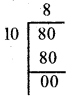2. $$\frac{7}{10}$$ = 0.7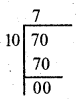3. $$\frac{6}{10}$$ = 0.6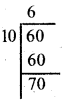4. $$\frac{16}{10}$$ = 1.6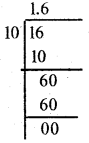5. $$\frac{42}{10}$$ = 4.2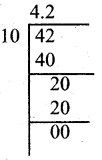6. $$\frac{83}{10}$$ = 8.3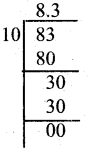7. $$\frac{7}{100}$$ = 0.07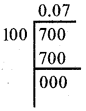8. $$\frac{72}{100}$$ = 0.72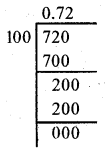9. $$\frac{861}{100}$$ = 8.61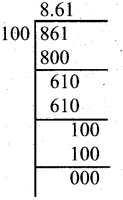10. $$\frac{162}{10}$$ = 16.2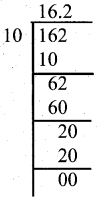11. $$\frac{141}{100}$$ = 1.41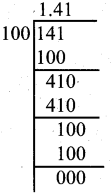12. $$\frac{1461}{100}$$ = 14.61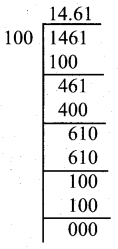13. $$\frac{1}{2}$$ = 0.5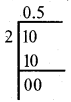14. $$\frac{3}{4}$$ = 0.75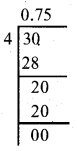15. $$\frac{2}{5}$$ = 0.4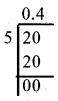16. $$\frac{7}{20}$$ = 0.35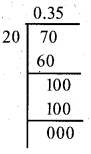17. $$\frac{38}{50}$$ = 0.76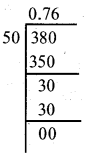18. $$\frac{17}{25}$$ = 0.68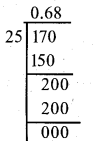19. $$\frac{13}{20}$$ = 0.65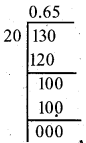20. $$\frac{8}{25}$$ = 0.32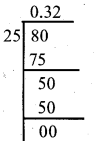II. Write the following decimal fractions as fractions:

1. 0.7
$$\frac{7}{10}$$

2. 0.02
$$\frac{2}{100}$$

3. 3.8
$$\frac{38}{10}$$

4. 14.5
$$\frac{145}{10}$$5. 0.56
$$\frac{56}{100}$$

6. 8.03
$$\frac{803}{100}$$

7. 14.57
$$\frac{1457}{100}$$

8. 85.4
$$\frac{854}{10}$$

9. 147.5
$$\frac{1475}{10}$$

10. 8.5
$$\frac{85}{10}$$

11. 85.61
$$\frac{8561}{100}$$

12. 6.84
$$\frac{8561}{100}$$